Shell Variables   «Prev  Next»
 Lesson 8 Using numbers in variables Objective Assign numbers to variables and manipulate them.

# Using Numbers in Variables

Numbers are assigned to shell variables in the same way that strings are assigned. For example, to assign the value “1492” to a variable named COUNT, use this statement:

```COUNT=1492
```

The shell does not track anything about the variable’s contents.
This variable is displayed like a number. If we use this assignment instead:
```YEAR=1492
```

then it is more like a string (a year) . But to the shell they are the same. This example does not use quotation marks because it contains no spaces, but the following assignment is equivalent:
```YEAR="1492"
```
The key to using variables as numbers is to make certain they contain only digits. This allows numeric operators to treat the variables as numbers.

### Evaluating expressions

The Bourne shell does not provide any way to evaluate an expression. Even something as simple as 5+7 cannot be calculated within a shell script unless you use an External Command.
You can see how this works with the `expr` command, which evaluates an expression:
External command: A command started from within a command interpreter (such as a UNIX shell) that is not part of the command interpreter's functionality, but must be launched as a separate (external) program.

```\$ expr 5 + 7
12
```

You can use the result of a command like this as the assigned value of a variable:
```TOTAL=‘expr 543 + 1983‘
```

The single back quotes cause the shell to execute the command between the quotes and substitute the resulting text in the line. The command
```expr 543 + 1983
```

returns a string of 2526, so the format above is equivalent to using this statement to assign a value to TOTAL:
```TOTAL=2526
```
You can do the same thing with variable values. The following statements assign values to two variables, then use the `expr` command to add those values together and assign the result to a third variable. The value is then printed to the screen using `echo`.

```FLIGHT=190
GROUND=45
TOTAL=‘expr \$FLIGHT + \$GROUND‘
```

The following Slide Show shows how the embedded command in this example is processed.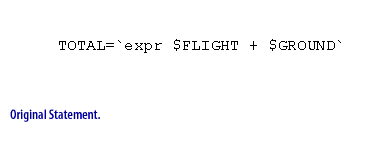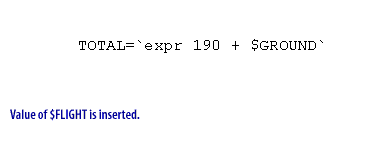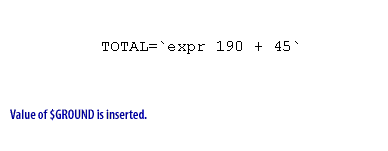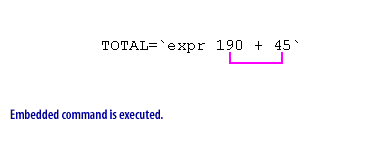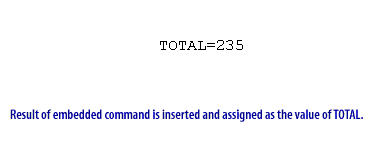embedded Command - Execution
The next course in the UNIX Shell Programming Series describes in detail how to use embedded commands (also called command substitution). If you use the bash, ksh or tcsh shells instead of sh, you can directly use numeric expressionsin your scripts, without using the `expr` command. For example, the following statement (which does not use any external commands) is valid in the bash shell:
```TOTAL_TIME=\$((\$FLIGHT_TIME + \$GROUND_TIME))
```

Using other operators to create expressions is the subject of the next lesson.